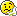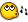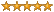## Recommended Posts

Hey all, I need to write a script to shut down a java.exe process. The problem is I can't use a shotgun and take out any and all java.exe processes, I need to kill the one owned by a particular user (dummy batch process id)

Looking at the available functions I don't see a way to access that information. A forum search was unenlightening.

Thoughts?

##### Share on other sites

Hey all, I need to write a script to shut down a java.exe process. The problem is I can't use a shotgun and take out any and all java.exe processes, I need to kill the one owned by a particular user (dummy batch process id)

Looking at the available functions I don't see a way to access that information. A forum search was unenlightening.

Thoughts?

I helped work on a function to get the full path to the exe file, could you get the file information you need by the file/folder location?

Edit:

Here's the link just in case: http://www.autoitscript.com/forum/index.ph...ndpost&p=165980

Larry actually has wrote the exe full path part I believe.

Edited by SmOke_N

Common sense plays a role in the basics of understanding AutoIt... If you're lacking in that, do us all a favor, and step away from the computer.

##### Share on other sites

Yup that would suffice!

##### Share on other sites

Yup that would suffice!

Common sense plays a role in the basics of understanding AutoIt... If you're lacking in that, do us all a favor, and step away from the computer.

##### Share on other sites

mmm works nicely except, unlike the topic of that thread, the process I need access to is not visible as it is a background java process, no window....Perhaps I can massage it..

##### Share on other sites

mmm works nicely except, unlike the topic of that thread, the process I need access to is not visible as it is a background java process, no window....Perhaps I can massage it..

The core functions you need to look at are the comments ----------Larry's code below--------- he actually wrote exactly what you need, and you can just mod the functions from there... maybe something like ProcessLists('Java.exe') and go throught only the java exe's that you need... let me know if you need a hand with it... could probably whip it up in a few minutes for ya.

Common sense plays a role in the basics of understanding AutoIt... If you're lacking in that, do us all a favor, and step away from the computer.

##### Share on other sites

Had an obvious error in my last post, so here is a script that will look for a process running from a specified directory and terminate it. This version only deals with java.exe processes though...

;

$killMe = "path\to\my\process.exe"$grossProcessList = _runningEXE()
$myProcessList = '' For$i = 1 To UBound($grossProcessList) - 1 if StringInStr($grossProcessList[$i], "java.exe") then$myProcessList = $myProcessList &$grossProcessList[$i] & @LF endIf Next ; local$myProcessArray = StringSplit($myProcessList, @LF) For$i = 1 to UBound($myProcessArray) - 1 If StringInStr($myProcessArray[$i],$killMe) Then
local $thisProcess =$myProcessArray[$i] local$myPIDstring = StringSplit($thisProcess, "|") local$myPID = $myPIDstring ProcessClose($myPID)
endIf
Next

;

Func _runningEXE()
Local $a_ProcessList = ProcessList() Local$a_DummyProcessList
For $iProcessList = 1 To$a_ProcessList
$a_DummyProcessList =$a_DummyProcessList & GetProcessName($a_ProcessList[$iProcessList]) & "|" & $a_ProcessList[$iProcessList] & Chr(01)
Next
Return StringSplit(StringTrimRight($a_DummyProcessList, 1), Chr(01)) EndFunc ; Func GetProcessName(ByRef$PID)
Local $Process,$Modules, $Ret Const$PROCESS_QUERY_INFORMATION = 0x0400
Const $PROCESS_VM_READ = 0x0010$Process = DLLCall("kernel32.dll","hwnd","OpenProcess","int", _
BitOR($PROCESS_QUERY_INFORMATION,$PROCESS_VM_READ),"int",0,"int",$PID) If$Process = 0 Then Return SetError(1)

$Modules = DLLStructCreate("int") DLLCall("psapi.dll","int","EnumProcessModules","hwnd",$Process,"ptr",DllStructGetPtr($Modules), _ "int",DllStructGetSize($Modules),"int_ptr",0)

$Ret = DLLCall("psapi.dll","int","GetModuleFileNameEx","hwnd",$Process,"int",DllStructGetData($Modules,1), _ "str","","int",2048)$Modules = 0
If StringLen($Ret) = 0 Then Return SetError(1) Return$Ret
EndFunc

;

##### Share on other sites

thanks alot. this helped me alot

##### Share on other sites

If just the process name known, then try this function (i am put together a couple functions from this forum and wrote one of my owne):

_ProcessCloseByOwner("Java.exe", "All")

Func _ProcessCloseByOwner($sProcess,$Owner)
If Not ProcessExists($sProcess) Then Return SetError(1) Local$i = 1, $PrcObj = "",$ExitLoop = 0, $Pid,$ExecPath, $objItem Local$objWMIService = ObjGet("winmgmts:\\" & @ComputerName & "\root\CIMV2")
Local $PrcObj =$objWMIService.ExecQuery ("SELECT * FROM Win32_Process", "WQL", 0x30)
Local $ProcList = ProcessList($sProcess)

While 1
$Pid =$ProcList[$i] If IsObj($PrcObj) Then
For $objItem In$PrcObj
If Int($objItem.ProcessId) =$Pid Then
$ExecPath =$objItem.ExecutablePath
If Not $ExecPath Then$ExecPath = _PathSearch($objItem.Caption) If FileExists($ExecPath) Then
If _GetExtProperty($ExecPath, 8) =$Owner Then
ProcessClose($Pid) If ProcessExists($Pid) Then ProcessClose($objItem.Caption)$ExitLoop = 1
ExitLoop
EndIf
EndIf
EndIf
Next
EndIf
$i += 1 If$ExitLoop Or $i >=$ProcList Then ExitLoop
WEnd
EndFunc

Func _GetExtProperty($sPath,$iProp)
Local $iExist,$sFile, $sDir,$oShellApp, $oDir,$oFile, $aProperty,$sProperty
$iExist = FileExists($sPath)
If $iExist = 0 Then SetError(1) Return 0 Else$sFile = StringTrimLeft($sPath, StringInStr($sPath, "\", 0, -1))
$sDir = StringTrimRight($sPath, (StringLen($sPath) - StringInStr($sPath, "\", 0, -1)))
$oShellApp = ObjCreate ("shell.application")$oDir = $oShellApp.NameSpace ($sDir)
$oFile =$oDir.Parsename ($sFile) If$iProp = -1 Then
Local $aProperty For$i = 0 To 34
$aProperty[$i] = $oDir.GetDetailsOf ($oFile, $i) Next Return$aProperty
Else
$sProperty =$oDir.GetDetailsOf ($oFile,$iProp)
If $sProperty = "" Then Return 0 Else Return$sProperty
EndIf
EndIf
EndIf
EndFunc

Func _PathSearch($ProcName) Local$PathArr = StringSplit(EnvGet("PATH"), ";"), $iN,$CurrentSearchPath
If IsArray($PathArr) Then For$iN = 1 To $PathArr$CurrentSearchPath = $PathArr[$iN] & "\" & $ProcName If FileExists($CurrentSearchPath) Then Return $CurrentSearchPath Next EndIf Return$ProcName
EndFunc

Spoiler

Using OS: Win 7 Professional, Using AutoIt Ver(s): 3.3.6.1 / 3.3.8.1

My Work...

SpoilerProjects:UDFs:Examples:

Like the Projects/UDFs/Examples? Please rate the topic (up-right corner of the post header: Rating)

* === My topics === *

====================================================================================================

AutoIt is simple, subtle, elegant. © AutoIt Team

## Create an account

Register a new account

• ### Recently Browsing   0 members

×

• Wiki

• Back

• #### Beta

• Git
• FAQ
• Our Picks
×
• Create New...